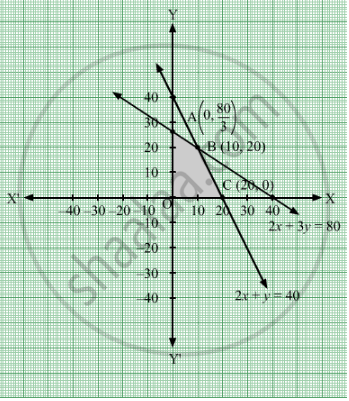# A Small Manufacturer Has Employed 5 Skilled Men and 10 Semi-skilled Men and Makes an Article in Two Qualities Deluxe Model and an Ordinary Model. - Mathematics

Sum

A small manufacturer has employed 5 skilled men and 10 semi-skilled men and makes an article in two qualities deluxe model and an ordinary model. The making of a deluxe model requires 2 hrs. work by a skilled man and 2 hrs. work by a semi-skilled man. The ordinary model requires 1 hr by a skilled man and 3 hrs. by a semi-skilled man. By union rules no man may work more than 8 hrs per day. The manufacturers clear profit on deluxe model is Rs 15 and on an ordinary model is Rs 10. How many of each type should be made in order to maximize his total daily profit.

#### Solution

Let articles of deluxe model and y articles of an ordinary model be made.
Number of articles cannot be negative.
Therefore, $x, y \geq 0$

According to the question, the making of a deluxe model requires 2 hrs. work by a skilled man and the ordinary model requires 1 hr by a skilled man

$2x + y \leq 40$
The making of a deluxe model requires 2 hrs. work by a semi-skilled man ordinary model requires 3 hrs. work by a semi-skilled man.
$2x + 3y \leq 80$
Total profit = Z = $15x + 10y$ which is to be maximised
Thus, the mathematical formulat​ion of the given linear programmimg problem is
Max Z = $15x + 10y$
subject to
$2x + y \leq 40$
$2x + 3y \leq 80$
$x \geq 0$
$y \geq 0$
The feasible region determined by the system of constraints isThe corner points are A(0, $\frac{80}{3}$B(10, 20), C(20, 0)

The values of Z at these corner points are as follows

 Corner point Z= 15x+10y A $\frac{800}{3}$ B 350 C 300

The maximum value of Z is 300 which is attained at C(20, 0)

Thus, the maximum profit is Rs 300 obtained when 10 units of deluxe model and 20 unit of ordinary model is produced
Concept: Graphical Method of Solving Linear Programming Problems
Is there an error in this question or solution?

#### APPEARS IN

RD Sharma Class 12 Maths
Chapter 30 Linear programming
Exercise 30.4 | Q 6 | Page 51